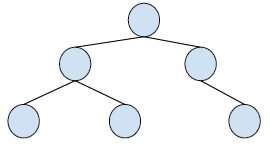# Print Common Nodes in Two Binary Search Trees in C++

C++Server Side ProgrammingProgramming

#### C in Depth: The Complete C Programming Guide for Beginners

45 Lectures 4.5 hours

#### Practical C++: Learn C++ Basics Step by Step

Most Popular

50 Lectures 4.5 hours

#### Master C and Embedded C Programming- Learn as you go

66 Lectures 5.5 hours

In this problem, we are given two binary search trees and we have to find the nodes that are common.

Binary Tree is a special tree whose every node has at max two child nodes. So, every node is either a leaf node or has one or two child nodes.

Example,Here, we have two binary trees and we have to print all the nodes that are the same for both trees.

Let’s create a program that uses an auxiliary stack to find the solution to this problem. It works by popping elements when two same values arise.

## Example

Live Demo

#include<iostream>
#include<stack>
using namespace std;
struct Node{
int key;
struct Node *left, *right;
};
Node *newNode(int ele){
Node *temp = new Node;
temp->key = ele;
temp->left = temp->right = NULL;
return temp;
}
void printCommon(Node *tree1, Node *tree2){
stack<Node *> stack1, s1, s2;
while (1){
if (tree1){
s1.push(tree1);
tree1 = tree1->left;
}
else if (tree2){
s2.push(tree2);
tree2 = tree2->left;
}
else if (!s1.empty() && !s2.empty()){
tree1 = s1.top();
tree2 = s2.top();
if (tree1->key == tree2->key){
cout << tree1->key << " ";
s1.pop();
s2.pop();
tree1 = tree1->right;
tree2 = tree2->right;
}
else if (tree1->key < tree2->key){
s1.pop();
tree1 = tree1->right;
tree2 = NULL;
}
else if (tree1->key > tree2->key){
s2.pop();
tree2 = tree2->right;
tree1 = NULL;
}
}
else break;
}
}
void inorderTraversal(struct Node *root){
if (root){
inorderTraversal(root->left);
cout<<root->key<<" ";
inorderTraversal(root->right);
}
}
struct Node* insertNode(struct Node* node, int key){
if (node == NULL) return newNode(key);
if (key < node->key)
node->left = insertNode(node->left, key);
else if (key > node->key)
node->right = insertNode(node->right, key);
return node;
}
int main(){
Node *tree1 = NULL;
tree1=insertNode(tree1, 45);
tree1=insertNode(tree1, 87);
tree1=insertNode(tree1, 12);
tree1=insertNode(tree1, 54);
tree1=insertNode(tree1, 89);
tree1=insertNode(tree1, 19);
tree1=insertNode(tree1, 72);
cout<<"Binary Tree 1 : ";
inorderTraversal(tree1);
cout<<endl;
Node *tree2=NULL;
tree2=insertNode(tree2, 72);
tree2=insertNode(tree2, 23);
tree2=insertNode(tree2, 13);
tree2=insertNode(tree2, 1);
tree2=insertNode(tree2, 19);
cout<<"Binary Tree 2 : ";
inorderTraversal(tree2);
cout<<endl;
cout<<"Common Nodes between the two trees : ";
printCommon(tree1, tree2);
return 0;
}

## Output

Binary Tree 1 : 12 19 45 54 72 87 89
Binary Tree 2 : 1 13 19 23 72
Common Nodes between the two trees : 19 72